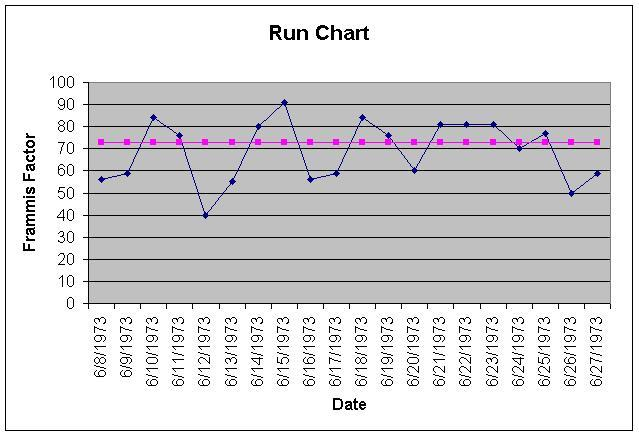# Run Chart

A run chart is a line graph that shows the changes in a data series over time.Typically, a run chart has two axes. Time is plotted on the horizontal axis; the vertical axis represents the value of the data point at a time of interest.

A second horizontal line is drawn at the median; half of the points are above and half of the points are below this line. This line is drawn from baseline data, which should be free of shifts, trends, runs, or astronomical data points.

Run charts are widely used in medical research, as well as in performance control and engineering applications.

A run chart makes it easy to see if there is a general degradation or improvement in the data series over time, or if the values you have are uncorrelated to time. If the only thing going on is random variations, you would expect to see the points scattered randomly above and below the median. A process of degradation might have the data series well above the median and slowly descending. A process of improvement might show a series beginning well below the median, with a continuous and general trend upwards.

## Shifts

Six or more consecutive points, either all above or all below the median, are called a shift. If a value falls right on the median, it doesn’t either break or add to a shift. For example, if you had 20 points in a row with a value of “2”, there would be no shift. When analyzing you will want to skip those points and go on to the next.

## Trends

A trend is five or more values all going in the same direction. Two consecutive points sharing the same value count as just one point in identifying trends.

## Runs

A run is a series of five points on one side of the median. These points can be going up, down, or a combination of up and down, as long as they remain on one side of the median line.

## Astronomical Points

An astronomical point is one that is very different from the other data in the run chart; any observer would agree that it is unusual. Don’t confuse these outliers with the chart minimum and maximum, which appear on every chart.

## References

Melton, K. Creating Run Charts